# Forecast evaluation

## Intro

This vignette provides a short overview of the basics of forecast evaluation with the functions from the onlineforecast package. It follows up on the vignettes setup-data and setup-and-use-model, and continues the building load forecast modelling presented there. If something is introduced in the present text, but not explained, then have a look in the two preceding vignettes to find an explanation.

First Load the package, setup the model and calculate the forecasts:

``````# Load the package
library(onlineforecast)``````

Just start by:

``````# Keep the data in D to simplify notation
D <- Dbuilding
# Keep the model output in y (just easier code later)
# Generate time of day in a forecast matrix
D\$tday <- make_tday(D\$t, 0:36)``````

## Score period

### Score period

Set the `scoreperiod` as a logical vector to control which points will be included in score calculations.

Use it to exclude a burn-in period of one week:

``````# Set the score period
D\$scoreperiod <- in_range("2010-12-22", D\$t)``````

### Train period

One fundamental caveat in data-driven modelling is over-fitting a model. This can easily happen when the model is fitted (trained) and evaluated on the same data. There are essentially two ways of dealing with this: Penalize increased model complexity or divide into a training set and test set (cross-validation).

In most forecasting applications the easiest and most transparent approach is some cross-validation approach - many methods for dividing into sets have been suggested. For online forecasting it is luckily quite straight forward, when a model is fitted using a recursive estimation method, like the RLS. In each time step the following happens:

• Data for the new time point becomes available, both observations and new forecasts.

• The parameters in the model are updated.

• A new forecast is calculated.

Hence, those forecasts are only calculated based on past data, so there is no need for dividing into a training set and a test set!

However, the parameters (like the forgetting factor and low-pass filter coefficients) are optimized on a particular period, hence over-fitting is possible, however it’s most often very few parameters compared to the number of observations - so the it’s very unlikely to over-fit a recursive fitted model in this setup.

## Models

``````# Define a new model with low-pass filtering of the Ta input
model <- forecastmodel\$new()
model\$output = "y"
I = "lp(I, a1=0.9)",
mu = "one()")
I__a1 = c(0.6, 0.9, 0.99),
lambda = c(0.9, 0.99, 0.9999))
# Optimize the parameters, only on two horizons
kseqopt <- c(3,18)
rls_optim(model, D, kseqopt)
##     ----------------
##     Ta__a1  I__a1 lambda
##       0.90   0.90   0.99
##        k3   k18   sum
##     0.814 0.812 1.626
##     ----------------
##     Ta__a1  I__a1 lambda
##      0.901  0.900  0.990
##        k3   k18   sum
##     0.814 0.812 1.626

## ...output cropped``````

Fit for all horizons and see the fit summary:

``````# Forecast for all horizons
model\$kseq <- 1:36
# Fit with RLS
fit1 <- rls_fit(model\$prm, model, D)
##     ----------------
##     Ta__a1  I__a1 lambda
##      0.897  0.920  0.991
# See the summary of the fit
summary(fit1)
##
##     Output: y
##     Inputs: Ta = lp(Ta, a1=0.897)
##              I = lp(I, a1=0.92)
##              mu = one()
##
##     Regression parameters:
##          lambda = 0.991
##
##     Scoreperiod: 1656 observations are included.
##
##     RLS coeffients summary stats (cannot be used for significance tests):
##           mean     sd    min     max
##     Ta -0.1900 0.0400 -0.370 -0.0050
##     I  -0.0075 0.0056 -0.024  0.0031
##     mu  5.4000 0.2800  4.800  6.4000
##
##     RMSE:
##       k1   k2   k3   k4   k5   k6   k7   k8   k9  k10  k11  k12  k13  k14  k15  k16
##     0.81 0.81 0.81 0.81 0.81 0.82 0.82 0.82 0.82 0.82 0.82 0.81 0.81 0.81 0.81 0.81
##      k17  k18  k19  k20  k21  k22  k23  k24  k25  k26  k27  k28  k29  k30  k31  k32
##     0.81 0.81 0.81 0.81 0.81 0.81 0.81 0.82 0.82 0.83 0.83 0.83 0.83 0.83 0.83 0.82
##      k33  k34  k35  k36
##     0.82 0.82 0.82 0.82``````

Let us extend the model by adding a new input: A diurnal pattern comprised by Fourier series. It can simply be added to the current model object:

``````# Add a diurnal curve using fourier series
# Optimize the parameters
rls_optim(model, D, kseq=kseqopt)
##     ----------------
##     Ta__a1  I__a1 lambda
##       0.90   0.90   0.99
##        k3   k18   sum
##     0.734 0.741 1.475
##     ----------------
##     Ta__a1  I__a1 lambda
##      0.901  0.900  0.990
##        k3   k18   sum
##     0.734 0.741 1.475

## ...output cropped``````

Fit for all horizons and see the fit summary:

``````# Fit with RLS
fit2 <- rls_fit(model\$prm, model, D)
##     ----------------
##     Ta__a1  I__a1 lambda
##      0.921  0.871  0.989
# Check the fit
summary(fit2)
##
##     Output: y
##     Inputs: Ta = lp(Ta, a1=0.921)
##              I = lp(I, a1=0.871)
##              mu = one()
##              mu_tday = fs(tday/24, nharmonics=4)
##
##     Regression parameters:
##          lambda = 0.989
##
##     Scoreperiod: 1656 observations are included.
##
##     RLS coeffients summary stats (cannot be used for significance tests):
##                     mean     sd     min   max
##     Ta           -0.1800 0.0520 -0.5100 0.620
##     I            -0.0027 0.0066 -0.0490 0.073
##     mu            5.3000 0.2900  4.6000 6.900
##     mu_tday.sin1  0.1700 0.1700 -0.7300 1.900
##     mu_tday.cos1 -0.2400 0.2400 -1.0000 0.760
##     mu_tday.sin2 -0.1900 0.1200 -1.1000 0.240
##     mu_tday.cos2 -0.1100 0.0820 -0.7500 0.180
##     mu_tday.sin3  0.1200 0.0880 -0.1000 0.440
##     mu_tday.cos3  0.2300 0.1200  0.0047 0.740
##     mu_tday.sin4 -0.0110 0.0790 -0.2400 0.190
##     mu_tday.cos4 -0.1200 0.0920 -0.4100 0.092
##
##     RMSE:
##       k1   k2   k3   k4   k5   k6   k7   k8   k9  k10  k11  k12  k13  k14  k15  k16
##     0.73 0.73 0.73 0.73 0.73 0.74 0.74 0.74 0.74 0.74 0.74 0.74 0.74 0.74 0.74 0.74
##      k17  k18  k19  k20  k21  k22  k23  k24  k25  k26  k27  k28  k29  k30  k31  k32
##     0.74 0.74 0.74 0.74 0.75 0.75 0.75 0.75 0.76 0.76 0.76 0.76 0.76 0.76 0.76 0.76
##      k33  k34  k35  k36
##     0.76 0.76 0.76 0.76``````

Keep the forecasts for plotting and later analysis:

``````# Keep the forecasts from each model by just inserting them in the data.list
D\$Yhat1 <- fit1\$Yhat
D\$Yhat2 <- fit2\$Yhat``````

Plot the forecasts for the full score period:

``````# Plot to see the forecasts for the shortest and the longest horizon
plot_ts(subset(D,D\$scoreperiod), c("^y|^Yhat1","^y|^Yhat2"), kseq = c(1,36))``````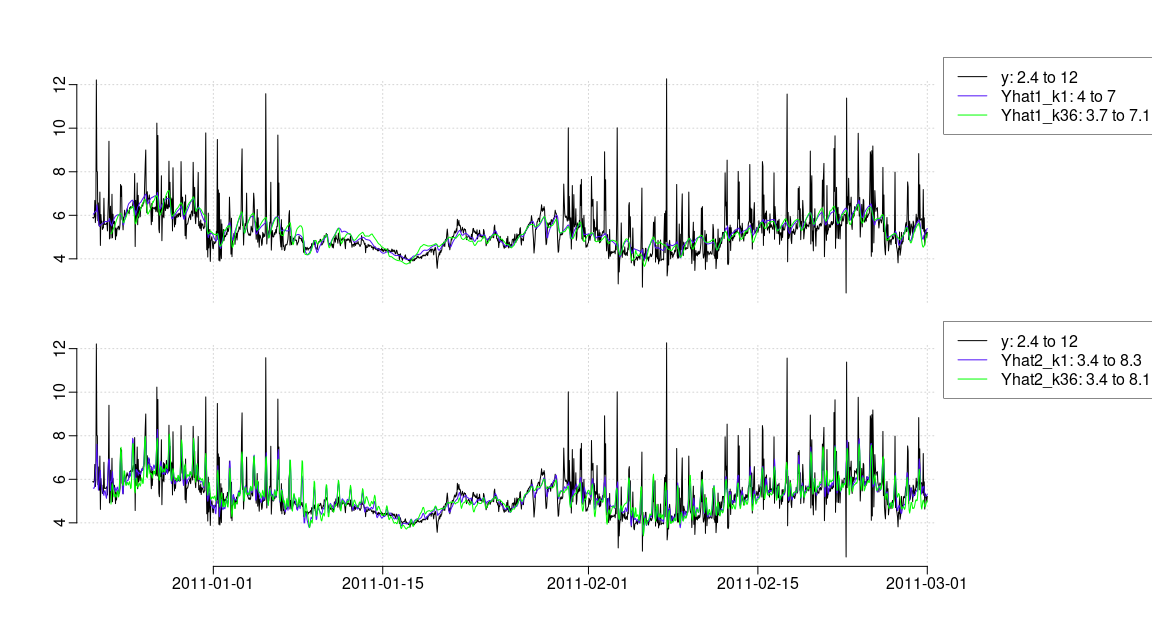Plot the full the first 14 days of the score period:

``````# Plot to see the forecasts for the shortest and the longest horizon
plot_ts(subset(D,which(D\$scoreperiod)[1:(14*24)]), c("^y|^Yhat1","^y|^Yhat2"), kseq = c(1,36))``````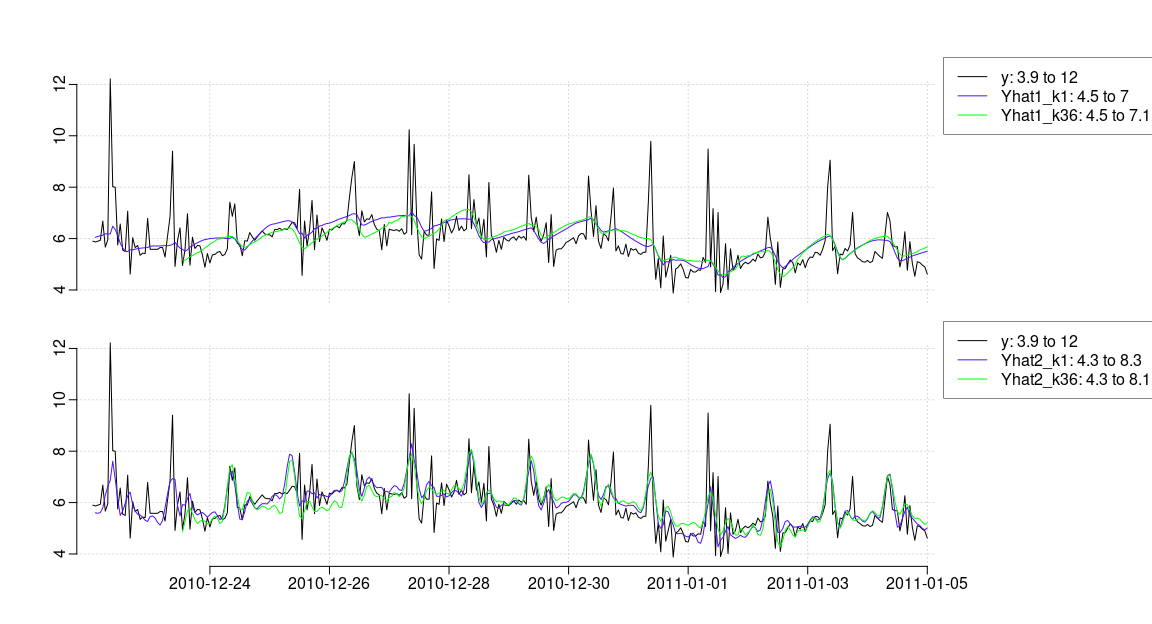We can see how adding the diurnal pattern enables to track the morning shower peaks.

## Reference models

The performance of a forecast model should be compared to a reference model. This is however not at all trivial, since the suitable reference model depends on the particular case of forecasting, e.g. the suitable reference model for wind power forecasting is not the same as for solar power forecasting - even within the same application the suitable reference model can be different depending on particular conditions etc.

In general the fundamental reference model should be the simplest reasonable model not relying on any inputs, hence either a model based on a mean calculation or some persistence should used. It can also be, that the study is about concluding the value of using NWPs as input, and in that case the reference model should be the best model without the NWPs.

We will here demonstrate how to generate persistence forecasts, both a persistence with the current model output and a diurnal persistence, which uses the latest value lagged a given period from the forecast time point.

First the simple persistence:

``````# The simple persistence (forecast for same horizons as the model)
D\$YhatP <- persistence(D\$y, model\$kseq)
# Plot a few horizons
plot_ts(D, c("^y\$|YhatP\$"), c("2011-01-05","2011-01-10"), kseq=c(1,24,36))``````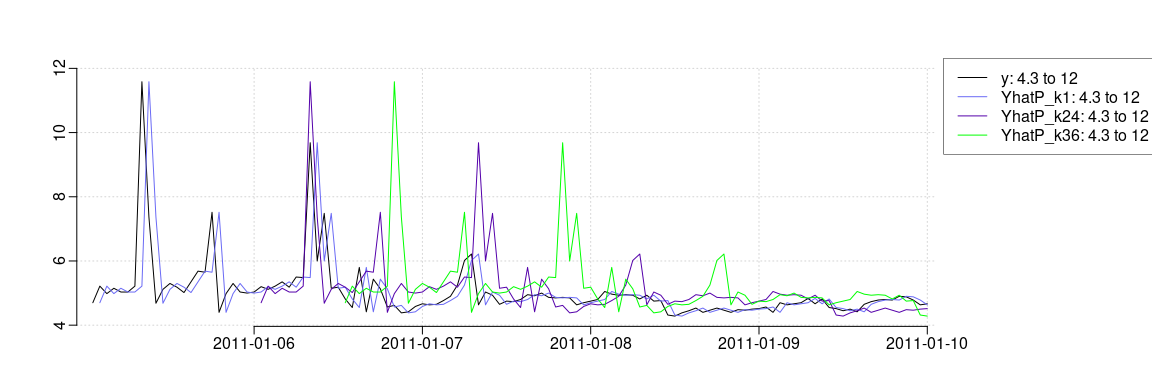Remember that the forecasts are lagged in the plot. Maybe it’s even more obvious to see that it’s simply the current value for all horizons:

``````D\$YhatP[1:4, 1:8]
##         k1   k2   k3   k4   k5   k6   k7   k8
##     1 5.92 5.92 5.92 5.92 5.92 5.92 5.92 5.92
##     2 5.85 5.85 5.85 5.85 5.85 5.85 5.85 5.85
##     3 5.85 5.85 5.85 5.85 5.85 5.85 5.85 5.85
##     4 5.88 5.88 5.88 5.88 5.88 5.88 5.88 5.88``````

A diurnal (i.e. 24 hours) persistence is: Take the value from the most recent time point, at the same time of day, as the forecast time point (i.e. `tod(t+k)`). It can be obtained by:

``````# Use the argument perlen to set the period length
D\$YhatDP <- persistence(D\$y, model\$kseq, perlen=24)
# Plot a few horizons
plot_ts(D, c("^y\$|YhatDP\$"), c("2011-01-05","2011-01-10"), kseq=c(1,24,36))``````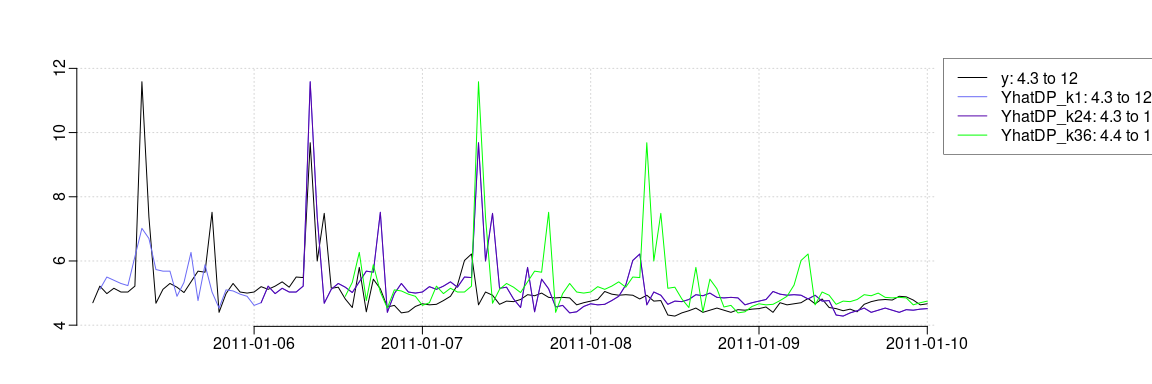Note how going beyond the `perlen` value, then the forecasts are the 48 hours lag values (going >48 the forecasts will be 72 lagged and so fourth).

## Score comparison

Now it’s just a matter of calculating the score, as a function of the horizon, for each model and compare them.

We have kept the forecasts in forecast matrices for each model, we can find them by:

``````# Find the forecasts in D
nms <- grep("^Yhat", names(D), value=TRUE)
nms
##      "Yhat1"  "Yhat2"  "YhatP"  "YhatDP"``````

So it’s the small and the large model, and as reference the simple and the diurnal persistence model.

One quite important point: When comparing forecasts from different models exactly the same forecast points must be included. When NAs are present, not all models predict the same values, e.g. a persistence model will leave forecasts after NAs, also as NAs.

So to make sure that exactly the same points are included in the score calculation, we must only forecast points where all forecasts are available (i.e. non-NA):

``````# Find all complete cases for all forecasts and horizons
ok <- complete_cases(D[nms])``````

Check if there are NAs in the forecasts:

``````sum(ok)
##      1708
length(ok)
##      1824``````

The forecasts will always have NAs from the start, e.g.:

``````D\$Yhat1[1:11, 1:10]
##           k1    k2    k3    k4     k5     k6      k7    k8   k9 k10
##     1     NA    NA    NA    NA     NA     NA      NA    NA   NA  NA
##     2   5.87    NA    NA    NA     NA     NA      NA    NA   NA  NA
##     3   5.87  5.82    NA    NA     NA     NA      NA    NA   NA  NA
##     4   5.89  5.85  5.87    NA     NA     NA      NA    NA   NA  NA
##     5   5.86  5.82  5.84  5.87     NA     NA      NA    NA   NA  NA
##     6   5.84  5.80  5.82  5.80  5.655     NA      NA    NA   NA  NA
##     7   5.90  5.85  5.83  5.73  5.550  5.332      NA    NA   NA  NA
##     8   8.13  7.66  7.21  6.37  7.220  7.400  7.2823    NA   NA  NA
##     9  10.49 22.99 29.41 27.65 16.401 13.794 13.2353 12.20   NA  NA
##     10  2.86  7.30  6.02  2.66  0.119  0.425  0.0766  7.03 4.51  NA
##     11  2.99  7.23  6.15  4.18  3.814  4.342  5.2472  5.95 3.87 3.3``````

but in this particular case other periods with NAs exists:

``````D\$y[59:72]
##        6.43 10.90    NA    NA    NA    NA    NA    NA    NA    NA    NA    NA
##       5.20  5.57
D\$YhatP[59:72, 1]
##        6.43 10.90    NA    NA    NA    NA    NA    NA    NA    NA    NA    NA
##       5.20  5.57``````

They have been excluded as non complete cases.

Actually we only want to include the scoreperiod in the evaluation:

``ok <- ok & D\$scoreperiod``

and there all models had complete forecasts:

``````sum(ok)
##      1656
sum(D\$scoreperiod)
##      1656``````

We can now use the `score()` function for calculating the score with only the complete cases found above:

``````# The score as a function of the horizon
R <- residuals(D\$Yhat1, D\$y)
score(R, ok & D\$scoreperiod)
##        k1    k2    k3    k4    k5    k6    k7    k8    k9   k10   k11   k12   k13
##     0.806 0.807 0.813 0.814 0.815 0.816 0.816 0.816 0.816 0.816 0.816 0.814 0.814
##       k14   k15   k16   k17   k18   k19   k20   k21   k22   k23   k24   k25   k26
##     0.814 0.814 0.813 0.812 0.812 0.813 0.811 0.810 0.809 0.810 0.815 0.820 0.826
##       k27   k28   k29   k30   k31   k32   k33   k34   k35   k36
##     0.829 0.829 0.827 0.826 0.825 0.824 0.823 0.823 0.823 0.822``````

Actually, the default way is to only use complete cases, hence:

``````# Only complete cases are used per default
score(R, D\$scoreperiod) == score(R, ok & D\$scoreperiod)
##       k1   k2   k3   k4   k5   k6   k7   k8   k9  k10  k11  k12  k13  k14  k15  k16
##     TRUE TRUE TRUE TRUE TRUE TRUE TRUE TRUE TRUE TRUE TRUE TRUE TRUE TRUE TRUE TRUE
##      k17  k18  k19  k20  k21  k22  k23  k24  k25  k26  k27  k28  k29  k30  k31  k32
##     TRUE TRUE TRUE TRUE TRUE TRUE TRUE TRUE TRUE TRUE TRUE TRUE TRUE TRUE TRUE TRUE
##      k33  k34  k35  k36
##     TRUE TRUE TRUE TRUE``````

Whether to use complete cases only can be controlled by:

``````# The score as a function of the horizon
score(R, usecomplete=FALSE) == score(R)
##        k1    k2    k3    k4    k5    k6    k7    k8    k9   k10   k11   k12   k13
##     FALSE FALSE FALSE FALSE FALSE FALSE FALSE FALSE FALSE FALSE FALSE FALSE FALSE
##       k14   k15   k16   k17   k18   k19   k20   k21   k22   k23   k24   k25   k26
##     FALSE FALSE FALSE FALSE FALSE FALSE FALSE FALSE FALSE FALSE FALSE FALSE FALSE
##       k27   k28   k29   k30   k31   k32   k33   k34   k35   k36
##     FALSE FALSE FALSE FALSE FALSE FALSE FALSE FALSE FALSE  TRUE``````

These steps can be made for all models by, where also only complete cases for all models are used per default:

``RMSE <- score(residuals(D[nms], D\$y), D\$scoreperiod)``

Plot the RMSE as a function of the horizon:

``````plot(0, type="n", xlim=range(model\$kseq), ylim=range(RMSE), xlab="Horizon k", ylab="RMSE (kW)")
for(i in 1:ncol(RMSE)){
points(model\$kseq, RMSE[ ,i], type="b", col=i)
}
legend("topleft", nms, lty=1, col=1:length(nms))``````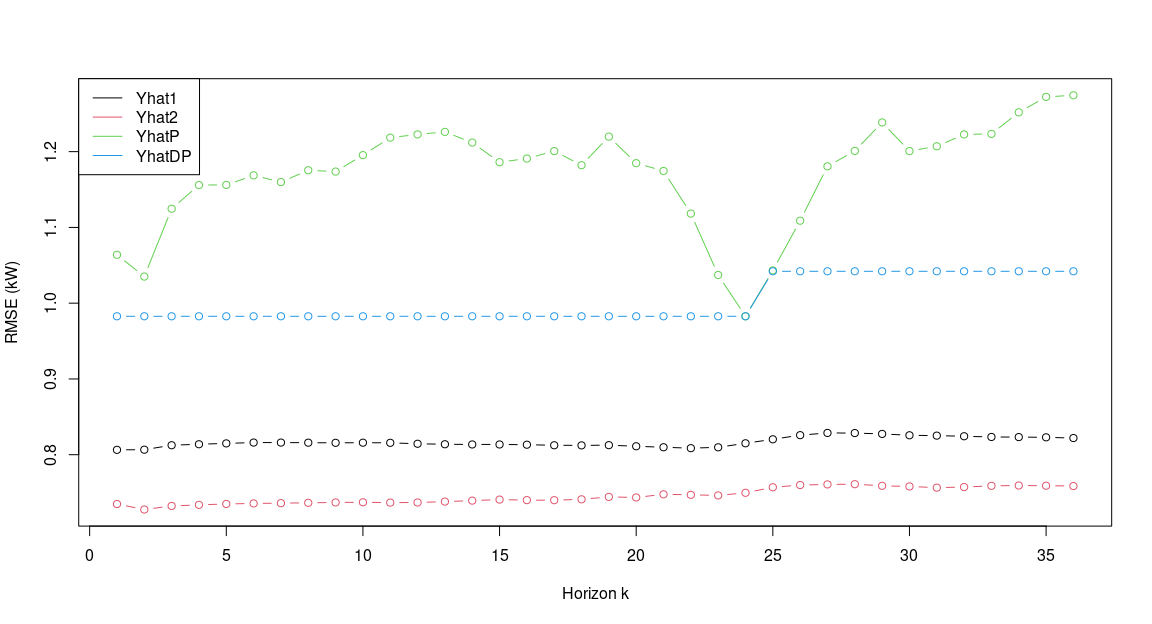### Training set and test set

As explained, it is most times not necessary to divide in a train and a test set when fitting recursively (i.e. using RLS), however it can sometimes be useful.

An easy approach is to set a logical vector, which is TRUE until the end of the training period (with a week of burn-in):

``````D\$trainperiod <- in_range(D\$t[7*24]-1, D\$t, "2011-02-01")
plot(D\$t, D\$trainperiod)``````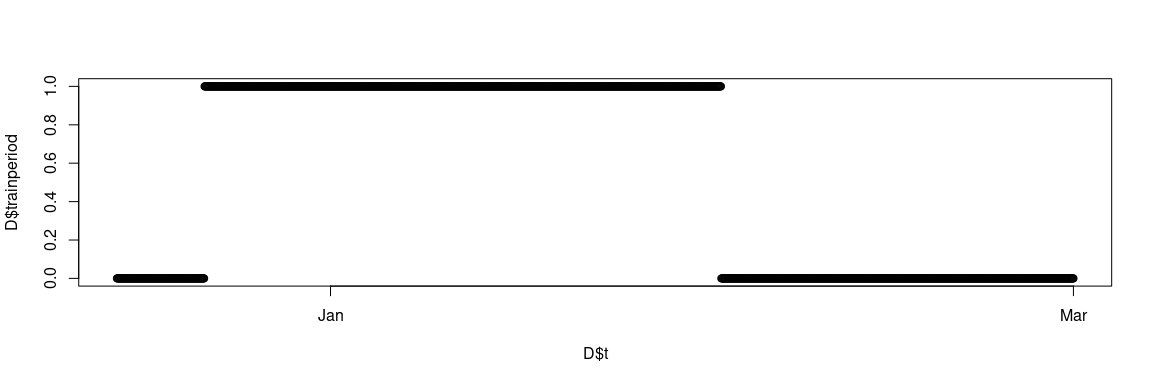then optimize the parameters only on this period, by taking a subset:

``````# Optimize the parameters
rls_optim(model, subset(D,D\$trainperiod), kseqopt)
##     ----------------
##     Ta__a1  I__a1 lambda
##       0.90   0.90   0.99
##        k3   k18   sum
##     4.927 0.911 5.839
##     ----------------
##     Ta__a1  I__a1 lambda
##      0.901  0.900  0.990
##        k3   k18   sum
##     4.927 0.911 5.838

## ...output cropped``````

and then fit on the entire set:

``````# Fit with RLS
fit <- rls_fit(model\$prm, model, D)
##     ----------------
##     Ta__a1  I__a1 lambda
##      0.946  0.600  0.908``````

Finally, the score can be calculated on the period following the train period by:

``````score(fit\$Yhat, !D\$trainperiod)
##       k1   k2   k3   k4   k5   k6   k7   k8   k9  k10  k11  k12  k13  k14  k15  k16
##     5.52 5.57 5.59 5.57 5.57 5.52 5.54 5.49 5.50 5.51 5.53 5.55 5.53 5.54 5.55 5.56
##      k17  k18  k19  k20  k21  k22  k23  k24  k25  k26  k27  k28  k29  k30  k31  k32
##     5.50 5.53 5.48 5.58 5.59 5.65 5.62 5.60 5.51 5.59 5.58 5.68 5.85 5.75 5.77 5.81
##      k33  k34  k35  k36
##     5.79 6.75 6.40 5.70``````

In this way it’s rather easy to set up different schemes, like optimizing the parameters once a week etc.

## Residual analysis and model validation

In the process of developing good forecasting models it is the always an interesting and informative experience (and necessary) to investigate the results of a model. Most of the time it boils down to investigating if there are any significant patterns left in the residuals - and how, if any, they can be described by extending the model.

Plot for the small model:

``````kseq <- c(1,18,36)
plot_ts(fit1, kseq=kseq)``````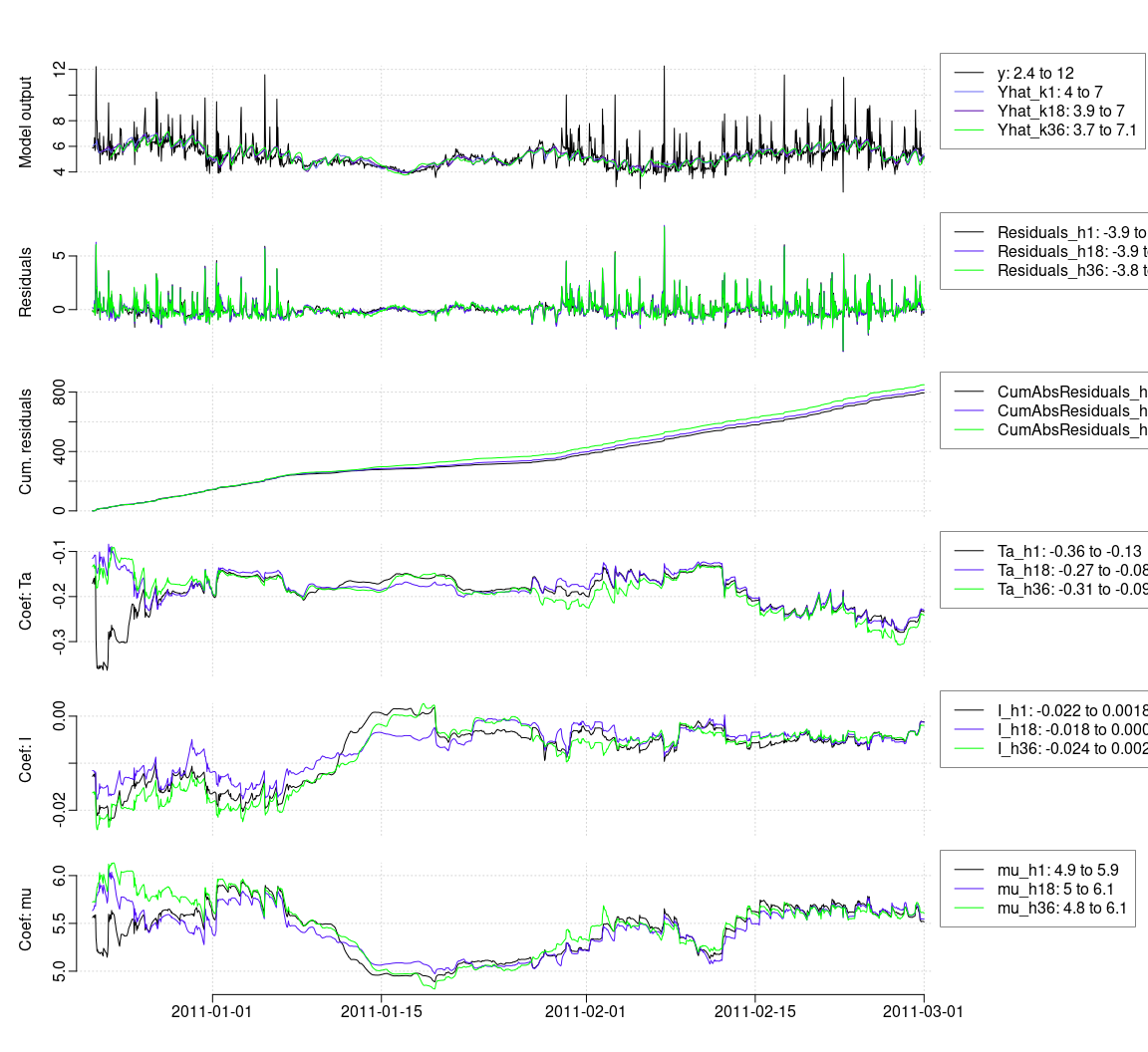In the second plot we see the residuals and it’s clear that there is a diurnal pattern - and in the lower three plots the coefficients has also a diurnal pattern.

Plot for the larger model (plots not included here):

``plot_ts(fit2, kseq=kseq)``

A pairs plot with residuals and inputs to see if patterns are left:

``````kseq <- c(1,36)
``````